- JoNova - http://joannenova.com.au -

Do greenhouse gases warm the planet by 33°C? Jinan Cao checks the numbers.

Posted By Joanne Nova On September 7, 2012 @ 4:36 am In Global Warming | Comments Disabled

Jinan Cao has been dissecting the nature of the greenhouse effect and a key calculation that I normally just accept without questioning. This will set a few pigeons loose, but it will be interesting to see where they land. The claims analyzed here are the oft repeated ones that the Earth’s greenhouse effect already warms us by 33°C and that a doubling of CO2 directly causes a 1.1°C rise (that’s with no feedbacks taken into account).

Jinan points out that these numbers, repeated as “fact”, are merely a result of misuse of the Stefan-Boltzmann equation. If Earth is not a perfect black body, but has an emissivity of 0.7 (as satellites suggest), then the temperature of the planet’s surface without any greenhouse effect would not be -18°C, but more like 5°C. That would mean the entire warming due to the greenhouse effect is only around 10°C, not the more impressive 33°C that is usually claimed. It means the greenhouse effect is probably less important than implied.

The 1.1°C direct rise that is predicted from doubling CO2 without feedbacks would also need to be recalculated. This paper does not try to do that, but if Jinan is right, that figure would be significantly lower too. Jinan looks at how that figure was derived. David Evans has been helping review Jinan’s work and writes the introduction below.  –  JoThis is the official IPCC version of the Earths Energy Budget as shown in the IPCC AR4 in 2007.

#### Introduction by Dr David Evans

The Stefan-Boltzmann equation describes how much radiation (energy) is given off by a body, such as a planet or a layer of air. It figures extensively in climate science, especially in simple calculations of where energy is flowing on average as the earth radiates heat into space as infrared radiation.

The Stefan-Boltzmann equation is used to obtain known nostrums of the climate debate, such as “it would be 33C cooler without greenhouse gases” and “the direct (no feedbacks) effect of doubling the CO2 levels is to raise the surface temperature by about 1.1C”. The equation is a standard one in physics. Its application to radiation from the earth can be done by any physicist or scientist; only a very rudimentary knowledge of climate science is required. (No mystical climate science secret knowledge relevant here; the laws of arithmetic and physics  are sufficient.)

Jinan Cao clinically dissects several of these applications of the Stefan-Boltzmann equation and finds it has been sloppily applied. Do you think the errors in application by the climate science community work in the direction of understating, or overstating, the role of CO2? Jinan’s article, although technical, is not difficult.

This paper will ruffle a few feathers and deserves a fair hearing. It brings up valid points, and challenges the application of the SB equation that are, by everyone’s admission, somewhat unsatisfactory.

Today’s climate scientists will attempt to laugh off these criticisms by saying that their models are much more sophisticated and accurate than the crude 0-D models considered when applying the SB equation. (A 0-D model treats the earth as a point, with zero dimensions. A 1-D model takes account of one dimension, either height in the atmosphere or latitude. A 3-D model treats the earth as having latitude, longitude, and height.)

But there are two problems with this defense. The first is authority. We don’t know what goes on inside these models, so are we supposed to just take the word of their modelers, that they have got it right? The models are too opaque and not open to public scrutiny, so this amounts to argument by authority. As the motto of the Royal Society says, take the word of nobody. The second problem is that today’s climate science community have demonstrated a habit of exaggerating issues even as straightforward as death threats, so how might they handle issues as vital to their funding as the importance of greenhouse gases to our well-being?

————————————–

Common errors in the use of the Stefan-Boltzmann equation

Jinan Cao

1.       INTRODUCTION

Climate scientists frequently make technical errors in their use of the Stefan-Boltzmann equation.

The Stefan-Boltzmann equation is simple: a black-body object with surface temperature, T, emits energy per unit time and unit surface area, J, the energy flux density:

J = σ T4                                                                                                                           (1)

where σ is the Stefan-Boltzmann constant equal to 5.67 x 10-8 (W/m2K4).

When the Stefan-Boltzmann law is applied to the Earth-Atmosphere system, climate scientists often make one or more of these technical errors:

i)        a coefficient ε in the range 0 to 1, called emissivity should multiply the right hand side, but not when applied to objects that are not black bodies;

ii)      a failure to specify correctly the “surface” and “surface temperature” of the Earth-Atmosphere system;

iii)    a failure to specify whether or not a layer of air is a single object or a cluster of objects.

These errors can be easily demonstrated by examining several statements (methodologies) most popular in current climate research:

1) the 33°C greenhouse warming effect for the Earth;

2) the 390 W/m2 surface radiation in the Earth Energy Budget;

3) the 1˚C CO2 non-feedback climate sensitivity; and

4) the formula for emission by a layer of air.

### 2. THEORETICAL ANALYSIS 2.1 The 33°C greenhouse warming effect for the Earth

It is repeatedly stated that the average temperature of the Earth would be 33°C lower than today if there were no greenhouse gas warming effect [1-5].  33°C = 15°C – (-18°C).  The -18°C is obtained from equation of radiative equilibrium between the incoming flux from the Sun and the outgoing flux from the Earth:

pr2 (1- α) S0 = 4pr2 ε σ T4                                                                                (2)

where r is the radius of the Earth,  α (alpha) is the albedo of the Earth, and S0 (=1368 W/m2) is the solar constant representing the incoming solar radiation energy flux density.  The symbol ε is emissivity of the earth surface.

In much current climate research, ε is either missing or assumed to be 1.  Inserting the values of  α = 0.3 and ε = 1 into Eq. (2), and solving for T:

(K) @ 255 (K) @ -18°C                                                                                   (3)

By adopting ε = 1, however, we are assuming that the earth’s surface is a black-body surface, which is never true.  If ε is not 1, but 0.9, 0.8, 0.7 or 0.6, T would be -11.4°C, -3.6°C, 5.5°C or 16.5°C respectively.  The finding of -18°C is simply a result of a technical error.  In fact, the emissivity of the earth surface can be determined ε ≈ 0.7 from satellite outgoing radiation spectra.

The Earth’s mean near-surface air temperature, as measured by global weather stations, is around 15°C ( 288K).  N2 and O2, which are literally transparent bodies, constitute 99% of the air.  This 15°C near surface air temperature is simply a different physical quantity that can not be used to subtract -18°C.  White and transparent bodies emit nothing at any temperature.

This error originates from a misunderstanding of the word “surface” that is a symbolised conception of the Stefan-Boltzmann law.  If there is no atmosphere, the surface means the land and water ground surface of the Earth, and T represents the mean temperature of the ground surface.  If there is atmosphere that is all of nitrogen and oxygen, the surface is still the ground surface, and T still the mean temperature of the ground surface, regardless what the air temperature may be.  This is because nitrogen and oxygen are non-radiative (literally ε = 0 for transparent and white bodies).  0 multiplying anything leads to 0.

When we identify the whole Earth-Atmosphere system as an object, its surface and surface temperature are no longer straightforward, but have different values for different radiation wavelengths.  Over the absorption bands of water vapour and carbon dioxide (e.g. the absorption band 15 μm for CO2), the surface is a layer of air starting from the top of atmosphere (TOA) with thickness equal to absorption depth, while the “surface temperature” is the mean temperature of CO2 molecules within the air layer ( -50°C). Similarly one can discover the surface and surface temperature for any other absorbing bands of radiative gases. For the rest of bands, the surface and surface temperature are the ground surface and its mean temperature ( 12°C)

### 2.2 The 390 W/m2 surface radiation in the Earth Energy Budget

Figure 1 is a diagram shown in the IPCC fourth report (AR4) as an estimate of the Earth’s annual and global mean energy balance [8-13].

We examine the surface radiation 390 W/m2 leaving the earth ground surface, which is considered to correspond to a blackbody emission, p, at 15°C as per the Stefan-Boltzmann equation (1):

p = σT4 = 5.67 ´ 10-8 ´ (273.15 + 15)4 = 390.89 @ 390 (W/m2)                     (4)

Firstly, the earth ground is never a black-body.  Emissivity for the earth ground surface, εg, is omitted in Eq. (4).

Secondly, the Earth’s mean near-surface air temperature 15°C has been used.  N2 and O2 emit literally nothing at whatever temperatures.  The T in Eq. (4) must be the temperature of the earth ground surface, which is 285.04 K (11.89°C) [6, 7], as determined from outgoing spectroscopy measurements and simulations.  The ground surface radiation then reads:

p = εg σT4 = εg ´ 5.67 ´ 10-8 ´ 285.044 = εg ´ 374.29 (W/m2)             (5)

The emissivity of the earth ground surface, εg, is unlikely close to 1.0.  Black body is an abstraction of a physical concept, hardly any substance is a black body on the Earth.

One can easily understand why the ground surface of the Earth would not completely absorb the 324 W/m2 back radiation because it is never a black body surface.  As these two figures are wrong, many other figures shown on the earth emission tree are called into question.

### 2.3 The 1˚C CO2 non-feedback climate sensitivity

It is well accepted in current climate research that a doubling of CO2 by itself contributes about 1°C to greenhouse warming, known as CO2 non-feedback climate sensitivity, or CO2 direct climate sensitivity [14, 15].  The debate is about feedback; a positive feedback will lead to higher, a negative feedback to lower, overall climate sensitivity.

Let us examine how this statement has been derived.  The energy emitted by the Earth-Atmosphere system per unit time and unit surface area (radiative flux, aka forcing), F, is written: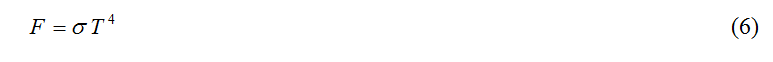The derivative of F with respect to T reads:Therefore,Eq. (8) has been interpreted to indicate how much warming DT occurs for any forcing increment.  If CO2 doubles, DF has been determined as 3.7 W/m2 by spectroscopic study.  Inserting DF =3.7 W/m2, T = 255 K into Eq. (8) leads to: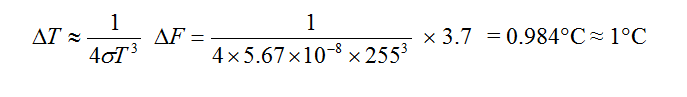Derivation including the emissivity reads,Taking advantage of the relationship between T and S0 in Eq. (2), one obtains:Inserting T = 255 K into Eq. (10) leads to the same answer of 0.985°C.  Note that DT depends on the emissivity ε via T, even though ε is not explicit in Eq. (10).  If ε is 1.0, 0.9, 0.8, 0.7 or 0.6, T would be -18°C, -11.4°C, -3.6°C, 5.5°C or 16.5°C respectively (as above), and, by Eq. (10),  would be 0.98°C, 1.01°C, 1.04°C, 1.07°C or 1.11°C respectively.

The error resulting from omission of emissivity thus cannot be more than 10%; it is more a methodological issue in this case.  The problem is, however, that the temperature T, is a physical quantity different from the Earth’s mean near-surface air temperature, Tair(h), which is largely the temperature of N2 and O2 that are literally transparent bodies emitting nothing at whatever temperatures.  The symbol h denotes altitude, almost 0 for near surface.  To estimate CO2 direct climate sensitivity, one must seek the relationship between DTair(h) ~ DF, not DT ~ DF.  There are heat transfer mechanisms other than radiation linking this T and Tair(h).

All the parameters must be consistent with the object defined either explicitly or implicitly.  The CO2 radiative forcing DF =3.7 W/m2 is actually the forcing of absorption by CO2 molecules in the atmosphere.  The outgoing forcing that leaves the Earth-Atmosphere object remains unchanged while doubling CO2, as long as the solar constant and albedo are unchanged.

### 2.4 The formula for emission by a layer of air

The atmosphere is from time to time represented by a layer (or layers) of air for climate modelling . Consider a given layer of air with temperature, Ta, and surface area, S, as shown in Figure 2. In current climate research the Stefan-Boltzmann Equation is straightforwardly applied to obtain σTa 4 for emitting energy flux density of the air layer. It is treated just like a sheet of solid (or condensed matter) object.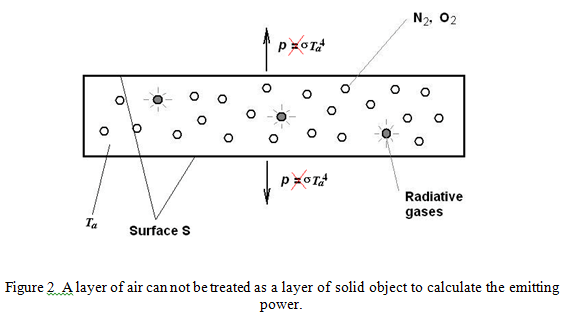As discussed above, N2 and O2 do not emit at any temperature.  Only the radiative gases in the air layer emit.  One will easily realise that i) only the temperature of radiative species is relevant instead of the average temperature of the layer – different gases may have different temperatures due to different radiation properties; ii) no gas is a black body, even the radiative gases.

There is a fundamental methodological error here.  Because the emitting species are so sparse in air, a given layer of air can not be identified as a single object applicable to the Stefan-Boltzmann law (strictly speaking, Planck’s law).  The correct methodology is to identify each single radiative molecule as an object that emits according to its temperature and radiative bands, forming a cluster of objects within the layer of air.  How much the layer of air emits must be determined by summing up all the radiation energy density emitted by each individual molecular object upon the surface S.  The principle of formulation is shown in a simple example as follows.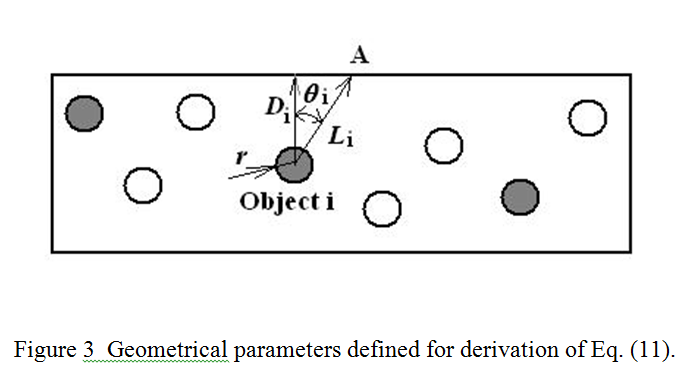Assume a layer of air containing n tiny spherical grey body objects with uniform radius, r, emissivity, ε, and uniform temperature T, the distance from each object to a given point on the surface of the air layer Li, where i is from 1 to n, as shown in Figure 3.  On the surface of each individual object, the emission flux density (energy per unit area and unit time) must follow the Stefan-Boltzmann equation, i.e. ε σT4.  The flux density decays with distance Li to (r/Li)2 ε σT4.  Therefore, at a given point on the surface of the air layer the overall flux density, p, reads: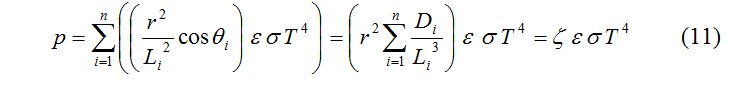where,is another coefficient (0 < ζ <= 1), which is missing in the current climate research.  Note ζ = 0 for n = 0, and ζ approaches 1 as n is sufficiently large enough in a given volume.  This coefficient applies as well for the Planck distribution function.

Eq. (11) indicates the flux density is very much depends on the number of objects within the air layer.  If the radiative objects are not dense enough within the air layer, the term of summation will be a very small fraction.  A strict mathematical expression can be formulised along this line but omitted in this article.

(This html version above may contain typos or errors as it was copied from the original. If in doubt, read the pdf!)

Jinan Cao is keen for feedback.

#### UPDATE: Michael Hammer writes a most well informed and thoughtful response at Comment #19

VN:F [1.9.22_1171]